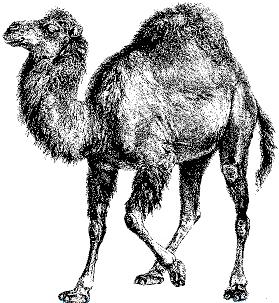# Nicholas Rinard Keene's Little Bit

I don't have a lot to say, but this is my little bit.

## Saturday, September 12, 2009

### Bubblesort in perlThis bubblesort sorts 3000 random integers between 1 and 1000 in 10 seconds.

```#!perl

use strict;

my (\$list_size, \$max_num, @numbers, \$start_time);

#Generate a large number of random integers
\$list_size = 3000;
\$max_num = 1000;
@numbers = ();
for(1..\$list_size) {
push(@numbers, int(rand(\$max_num)));
}

#Print out the number array, then sort it, then print it out again in sorted order
#print "\n\n@numbers";
\$start_time = time;
bubblesort(\@numbers);
#print "\n\n@numbers";
print "BUBBLESORT sorted \$list_size numbers between 1 and \$max_num in " . (time - \$start_time) . " seconds\n";

sub bubblesort {
my( \$numbers, \$swaps );
(\$numbers) = @_;
\$swaps = 0;

do {
\$swaps = 0;
for(my \$i=0; \$i < \$#{\$numbers}; \$i++) {
if ( \$numbers->[\$i] > \$numbers->[\$i + 1] ) {
swap( \$i, \$i+1, \$numbers);
\$swaps++;
}
}
} while( \$swaps > 0 );
}

#A swap operation takes a list reference and exchanges the values at the indicated indices
#The swap operation allows quicksort to work in one list
sub swap {
my( \$index1, \$index2, \$anyarray ) = @_;
\$_ = \$anyarray->[\$index1];
\$anyarray->[\$index1] = \$anyarray->[\$index2];
\$anyarray->[\$index2] = \$_;
}```